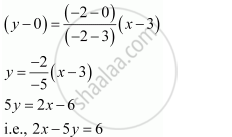# By Using the Concept of Equation of a Line, Prove that the Three Points (3, 0), (–2, –2) and (8, 2) Are Collinear. - Mathematics

By using the concept of equation of a line, prove that the three points (3, 0), (–2, –2) and (8, 2) are collinear.

#### Solution

In order to show that points (3, 0), (–2, –2), and (8, 2) are collinear, it suffices to show that the line passing through points (3, 0) and (–2, –2) also passes through point (8, 2).

The equation of the line passing through points (3, 0) and (–2, –2) isIt is observed that at x = 8 and y = 2,

L.H.S. = 2 × 8 – 5 × 2 = 16 – 10 = 6 = R.H.S.

Therefore, the line passing through points (3, 0) and (–2, –2) also passes through point (8, 2). Hence, points (3, 0), (–2, –2), and (8, 2) are collinear.

Concept: Various Forms of the Equation of a Line
Is there an error in this question or solution?

#### APPEARS IN

NCERT Class 11 Mathematics
Chapter 10 Straight Lines
Q 20 | Page 220